# Mann-Whitney Test for Two Samples HelpThe Mann-Whitney test is used when you want to determine if the results from one population or larger than the results from another population. For example, you might want to do if a group of patients treated with a new vaccine did not get ill as often as a group of untreated patients. The example below shows how to do this test using the SPC for Excel software. The median is once again used in this test since it is a better measure of the central tendency of the data than the average for non-normal data.

Example (from Statistics and Data Analysis by Ajit Tamhane and Dorothy Dunlop, Prentice-Hall, 2000)

The data below is the failure time for 18 capacitors. Eight had been tested under normal operating conditions; ten had been tested under thermally stress conditions.

Normal: 5.2, 8.5, 9.8, 12.3, 17.1, 17.9, 23.7, 29.8

Stress: 1.1, 2.3, 3.2, 6.3, 7, 7.2, 9.1, 15.2, 18.3, 21.1

We want to use these results to see if there is a difference between the two groups.

1. Enter the results into an Excel worksheet as shown below. The data can be downloaded at this link.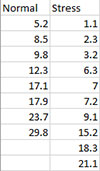2. Select the data including the headings.

3. Select “NonParametric” from the “Statistical Tools” panel on the SPC for Excel ribbon.

4. Select the “Mann-Whitney Test for Two Samples” option and then OK.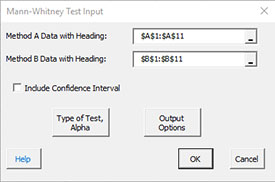• Method A Data with Heading: enter the range containing the data with heading; default is the range selected on the worksheet.
• Method A Data with Heading: enter the range containing the data with heading; default is the range next to Method A.
• Include Confidence Interval: check if you want the confidence interval displayed; default is not to display the confidence interval.
• Type of Test, Alpha: the input form below is displayed if this option is selected.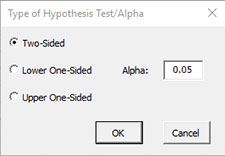• Select the Type of Test: two-sided; lower one-sided, or upper one-sided, the default is two-sided.
• Alpha: this is the confidence level; 1-alpha is the confidence interval, default is 0.05 or 95% confidence interval.
• Output Options: the input form below is displayed if this option is selected.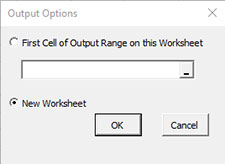• First Cell of Output Range on this Worksheet: Select this option if you want the output on an existing worksheet; then select the first cell of the range where you want the output placed; a check is made to ensure that no existing data are overwritten in the worksheet.
• New Worksheet: Select this option if you want the results on a new worksheet.
• Select OK to generate the results.
• Select Cancel to end the program.

Mann-Whitney Test for Two Samples Output

The output from the Mann-Whitney test is shown below.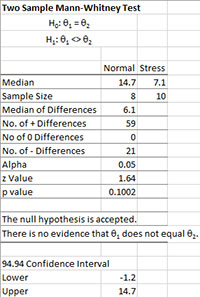The null hypothesis (m is median) and the alternate hypothesis (two sided in this example) are listed at the top.

• Median: Calculated medians of the two methods.
• Sample Size: Sample size of the two methods.
• Median of Differences: Median of the differences.
• No. of + Differences: Number of + differences for each pair of numbers.
• No of 0 Differences: Number where the pair of numbers are equal.
• No. of – Differences: Number of – differences for each pair of numbers.
• Alpha: Value of alpha entered by user.
• z Value: Calculated z value .
• p value: Calculated p value (will be red if ≤ alpha).

The conclusions, based on value of p and alpha, are given. Reject the null hypothesis if p value < alpha.

Notes:

• The software uses a normal approximation for this test.
• In the case of ties, 0.5 is added to both the + differences and the – differences.
• Exact confidence intervals cannot usually be calculated. The confidence interval given is two-sided and based on a normal distribution approximation with a continuity correction.